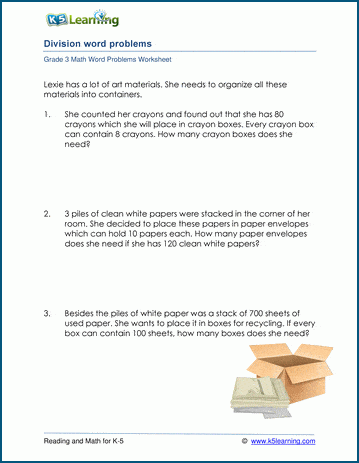# Division Word Problem Worksheets for 3rd Grade

## Third Grade Division Word Problem Worksheets

These math worksheets each have a number of simple simple division word problems.  After reading the word problem and understanding the 'real world scenario', the student must formulate the division equation to solve the problem.

## Long Division with remainders word problems

These grade 3 word problem worksheets require division "with remainders" to solve.  The numbers are relatively easy (numbers between 1 and 100).

We also have mixed multiplication and division word problems for grade 3 which students can try; mixing questions challenges students to fully understand the problem before applying a mechanical solution.Sample Grade 3 Division Word Problem Worksheet

## More word problem worksheets

Explore all of our math word problem worksheets, from kindergarten through grade 5.

## What is K5?

K5 Learning offers reading and math worksheets, workbooks and an online reading and math program for kids in kindergarten to grade 5.  We help your children build good study habits and excel in school.